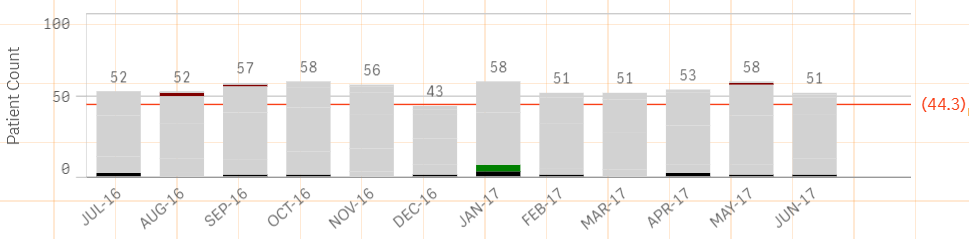New to Qlik Sense

Discussion board where members can get started with Qlik Sense.

Announcements
QlikWorld 2020: Join us May 11 - 14, 2020 in Phoenix, AZ. Register early and save \$400. Learn More
Highlighted
New Contributor III

Color consistency b/w Bar chart vs Pie Chart

Hi ,

I have a requirement like below.

I want to show the same color for Pie chart as well as bar chart.

Dimension for  Bar chart --  I used 2 dimensions (Month Name (Jan-17).... Dec-17 ,Days)

Days Dimension -- I have used

if([xyz] <=1, 'Below 1 day'

',if([xyz] <=3,  'Below 2-3 day'

',if([xyz] <=7,'Below 3-7 day'

',if([xyz] <=17,'Below  8-17 day'

',if([xyz] <=30, 'Below 18-30 day'

,above 30 days

)'))))

Measure - Used    Count([xyz])+ 0 * Count([xyz])

Same thing for pie chart also

I used color by expression in both charts

if([xyz] <=1, Yellow()

',if([xyz] <=3, blue()

',if([xyz] <=7, Green()

',if([xyz] <=17, brown()

',if([xyz] <=30, red()

,Red()

)'))))

But still it's not coming correctly. Kindly help me on this

Tags (3)
1 Solution

Accepted SolutionsMVP

Re: Color consistency b/w Bar chart vs Pie Chart

In essence, use the same set analysis that you use in your expression here

Only({<SetAnalysisFromYourExpression>}

if([xyz] <=1, Yellow()

',if([xyz] <=3, blue()

',if([xyz] <=7, Green()

',if([xyz] <=17, brown()

',if([xyz] <=30, red()

,Red()

)'))))

)

12 Replies
Valued Contributor

Re: Color consistency b/w Bar chart vs Pie Chart

I think you have to re write your if condition like you have to mention condition like,

if([xyz]<=1,yellow(),if([xyz]>1 and [xyz]<=3,blue(),if([xyz]>3 and [xyz]<=7,green())))

because in above condition there is confusion you are mentioning different colors for <=1,<=3 and so on,e.g. if your value is -1 then statement is true for both the conditionMVP

Re: Color consistency b/w Bar chart vs Pie Chart

Would you be able to share a sample to show the issue?

New Contributor III

Re: Color consistency b/w Bar chart vs Pie Chart

I have tied this too. but no luck

IF([Length of Stay] <= 1 ,  rgb(0,0,0),

If([Length of Stay] >1 AND  [Length of Stay] <=3,  Green(),

if(  [Length of Stay] >3  AND  [Length of Stay] <= 7 , Yellow(),

if(  [Length of Stay] >7  AND  [Length of Stay] <=17 , CYAN(),

if( [Length of Stay] >17  AND  [Length of Stay] <=30 , Red ()

, Magenta()

)))))

Valued Contributor

Re: Color consistency b/w Bar chart vs Pie Chart

Days dimension you have calculated on chart or at script level.

because if you have calculated at script then you can try condition like,if([xyz]='Below 1 day',blue(),if([xyz]='Below 2-3 day',yellow())) and so on.

New Contributor III

Re: Color consistency b/w Bar chart vs Pie Chart

Hi Sunny,

Here, I can't see the different colors for different categoryMVP

Re: Color consistency b/w Bar chart vs Pie Chart

Try this... I think the issue is that you might be making selections which might be causing this. I have used {1} to ignore all selections for your color expression

Only({1}

if([xyz] <=1, Yellow()

',if([xyz] <=3, blue()

',if([xyz] <=7, Green()

',if([xyz] <=17, brown()

',if([xyz] <=30, red()

,Red()

)'))))

)

Honored Contributor II

Re: Color consistency b/w Bar chart vs Pie Chart

The best way to manage colour consistently across your apps is to make your standard dimensions and measures master items and then use the built in feature to colour particular values.

Then when you create a chart of whatever type using those master items you can set it to use libray colours and your chart will use those master colours.

Regards

Andy

New Contributor III

Re: Color consistency b/w Bar chart vs Pie Chart

It's working partially.

Eg :

I am able to see the different color for different category.

ButIf select year = 2017 . i want to display only 12 months.

But in our expression.it pull all the months for all years rather than particular yearMVP

Re: Color consistency b/w Bar chart vs Pie Chart

Do you have a set analysis that you use in your main expression to see 12 months?